# Question 2 [10 points] Find an invertible matrix P and a diagonal matrix D such that...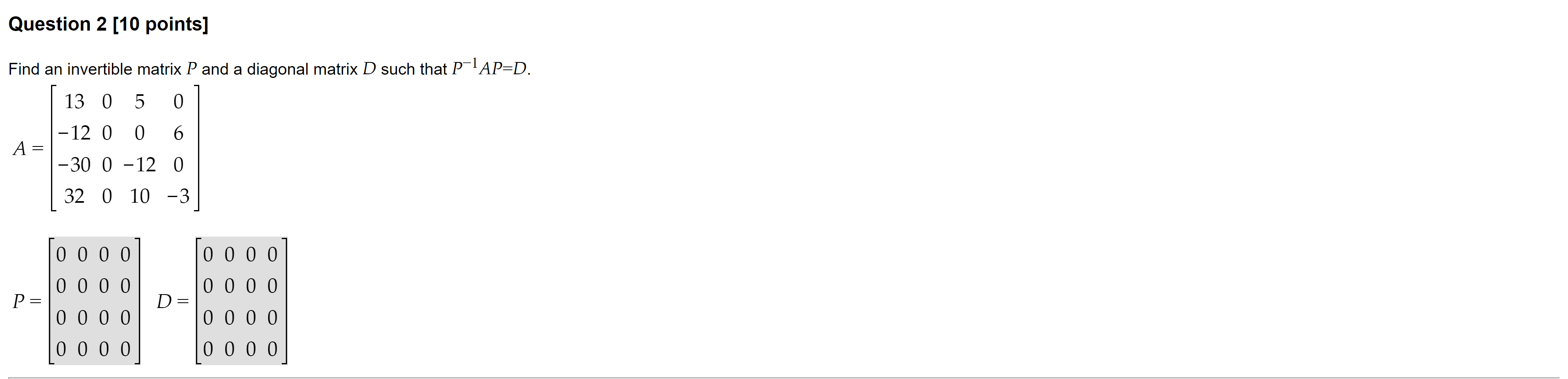Question 2 [10 points] Find an invertible matrix P and a diagonal matrix D such that P-AP=D. [ 13 0 5 0 -12 0 0 6 1-30 0 -12 0 | 32 0 10 -3) A = 1 P= To o o o 0 0 0 0 0 0 0 0 0 0 0 0 0 o o o o 0 0 0 0 0 0 0 0 0 0 0 0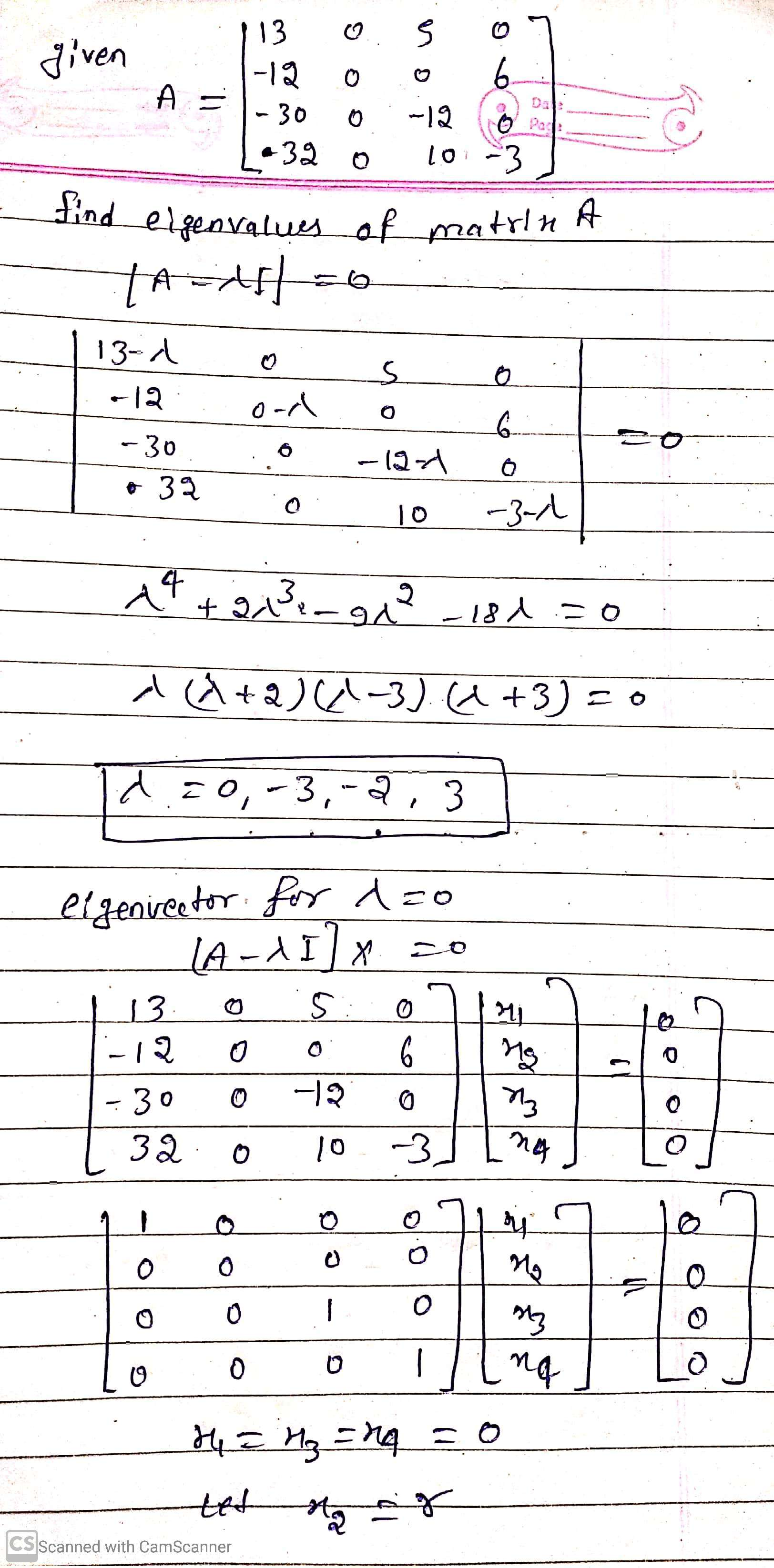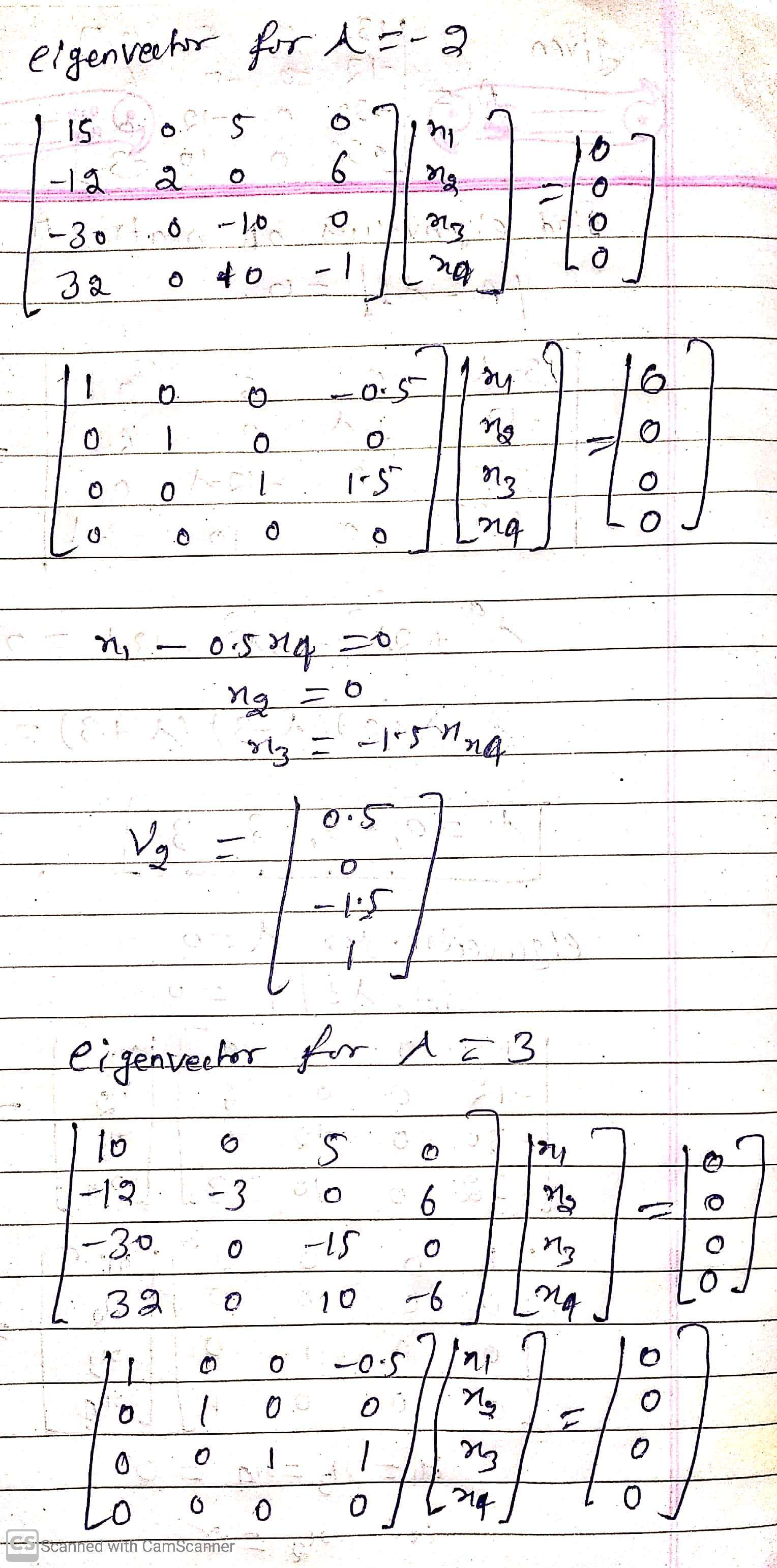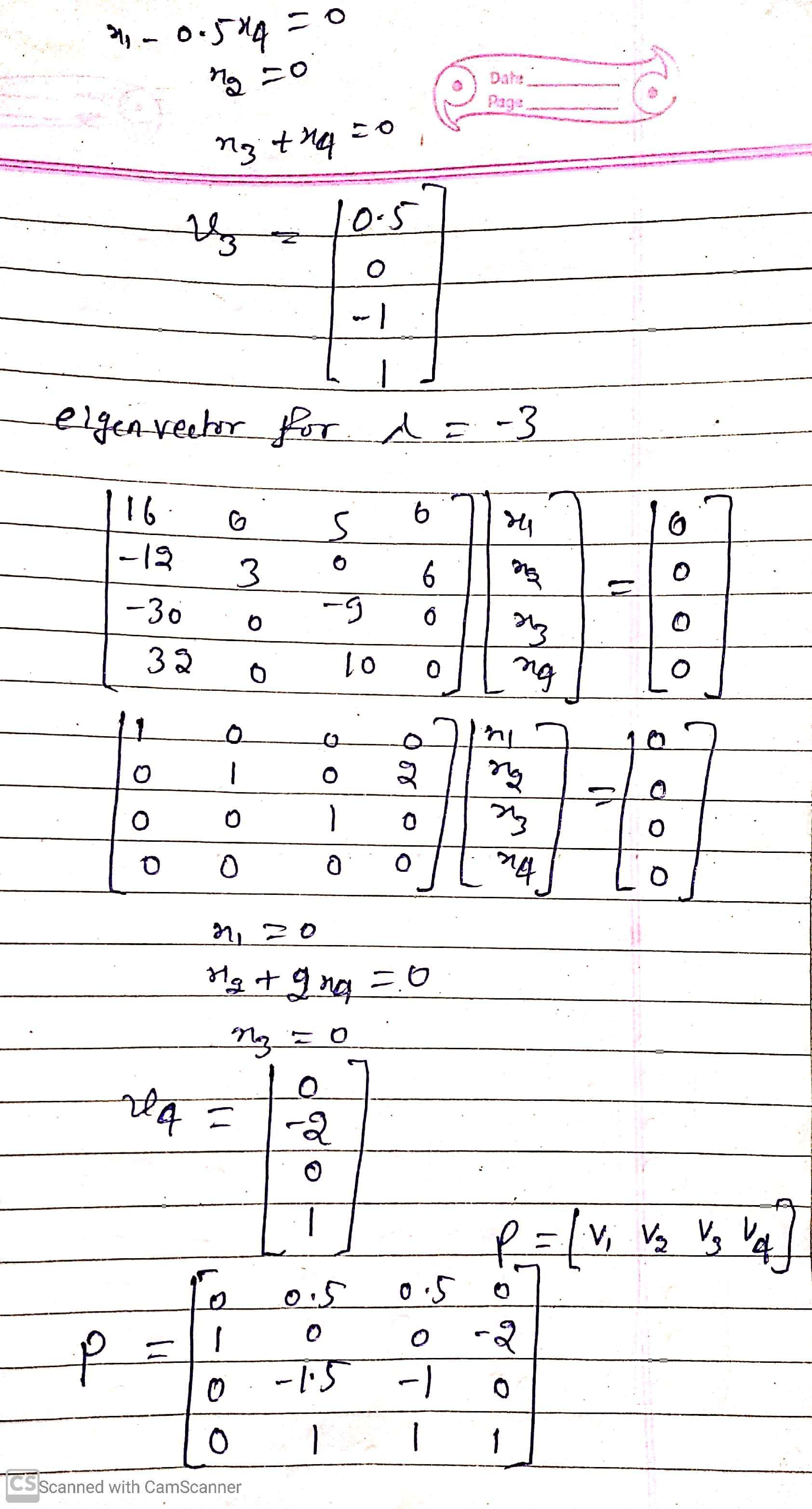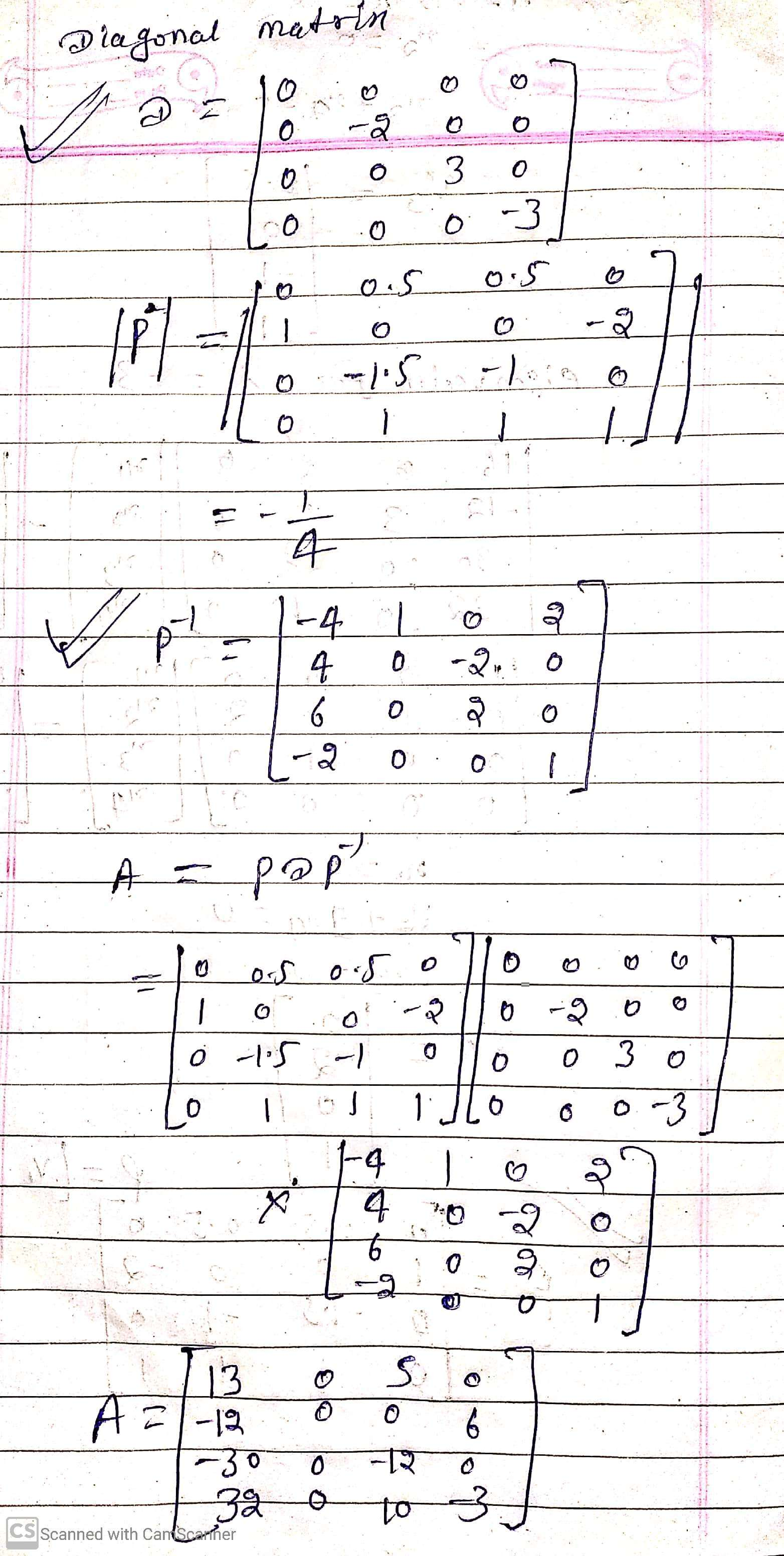#### Earn Coin

Coins can be redeemed for fabulous gifts.

Similar Homework Help Questions
• ### Question 12 [10 points] Find an invertible matrix P and a diagonal matrix D such that...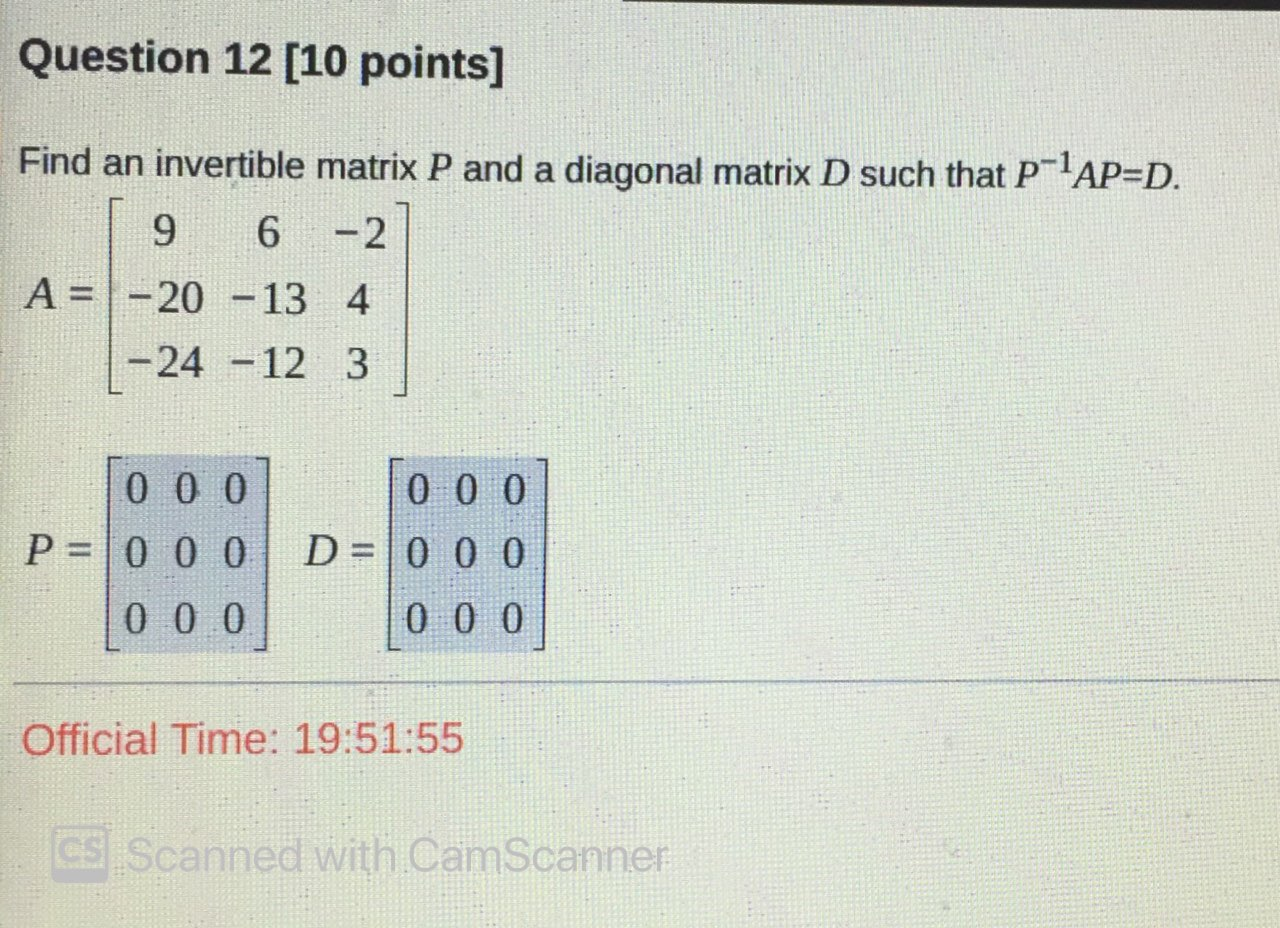Question 12 [10 points] Find an invertible matrix P and a diagonal matrix D such that P-lAP=D. | 9 6 -2 A= -20 -13 4 | -24 -12 3 Tooo oool P= 0 0 0 D = 0 0 0 0 0 0 | 0 0 0 Official Time: 19:51:55 CS Scanned with CamScanner

• ### Find an invertible matrix P and a diagonal matrix D such that P- AP-D -9 0...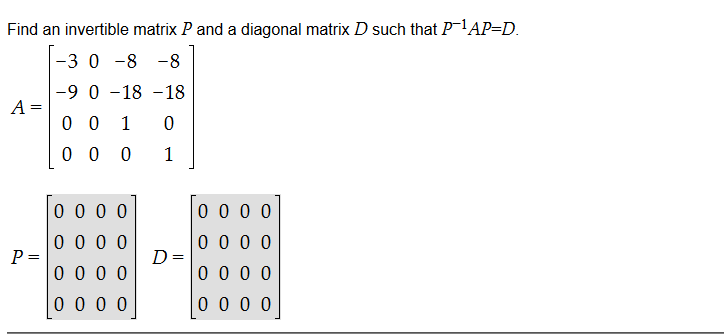Find an invertible matrix P and a diagonal matrix D such that P- AP-D -9 0 -18 -18 00 0 1 D D O O 0000| D=

• ### A. B. (1 pt) 1 0 Let/ = 184 Find an invertible matrix P and a diagonal matrix D such that PDPA D= (1 pt) 1 5 -15 LetA=1...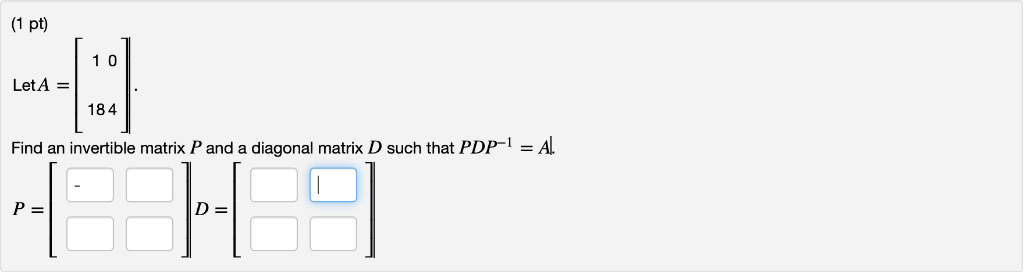A. B. (1 pt) 1 0 Let/ = 184 Find an invertible matrix P and a diagonal matrix D such that PDPA D= (1 pt) 1 5 -15 LetA=10-1 6 0-1 4 Find an invertible matrix P and a diagonal matrix D such that D = p- D= (1 pt) 1 0 Let/ = 184 Find an invertible matrix P and a diagonal matrix D such that PDPA D= (1 pt) 1 5 -15 LetA=10-1 6 0-1 4 Find an...

• ### 5.3: Diagonalization Find the diagonal matrix D and invertible matrix P such that A- PDp-1 if pos...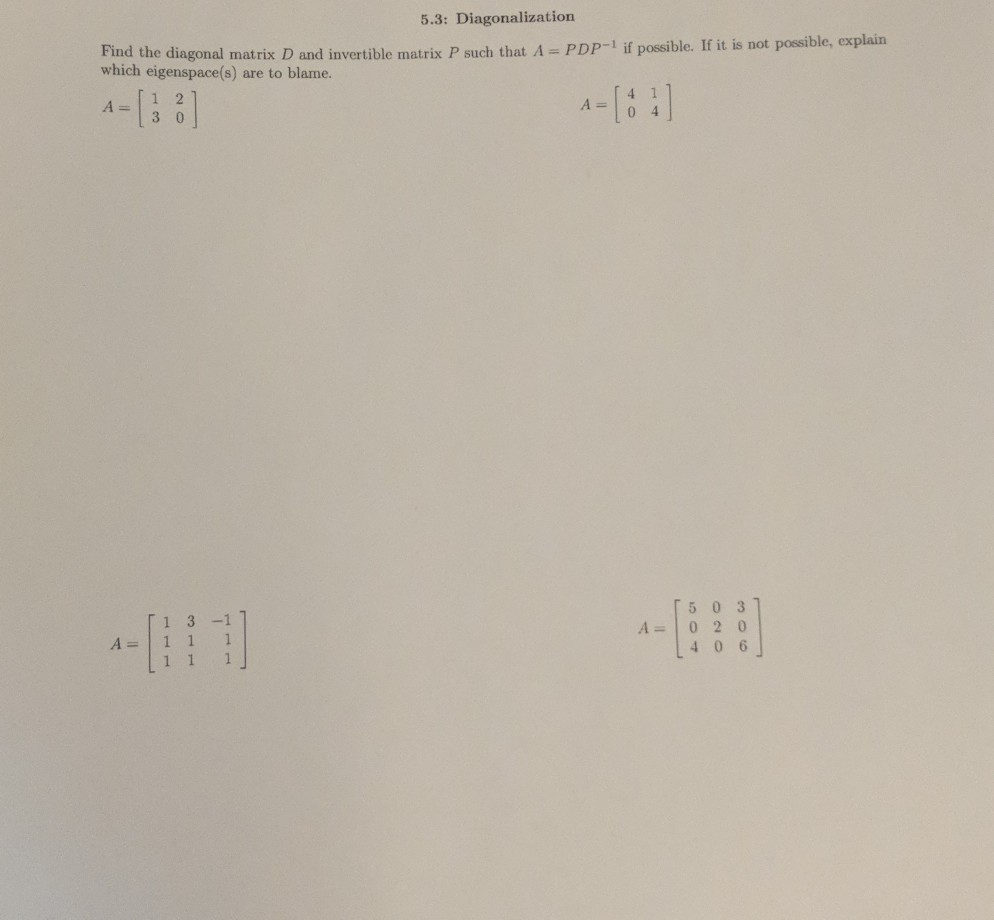I 5.3: Diagonalization Find the diagonal matrix D and invertible matrix P such that A- PDp-1 if possible. If it is not possibl which eigenspace(s) are to blame. e, eosplain A-1 2 1 3 -1 A 1 1 1 5 0 3 A- 0 2 0 し406 5.3: Diagonalization Find the diagonal matrix D and invertible matrix P such that A- PDp-1 if possible. If it is not possibl which eigenspace(s) are to blame. e, eosplain A-1 2 1 3...

• ### Diagonalize the matrix A. That is, find an invertible matrix P and a diagonal matrix D such that

Let A be the matrix4 -12 1Diagonalize the matrix A. That is, find an invertible matrix P and a diagonal matrix Dsuch thatP^-1AP = DFind P^-1.... Use the factorization A = PDP^-1 to compute A^5please explain

• ### Question 3 (1 point) Find an invertible matrix P and a diagonal matrix D that show...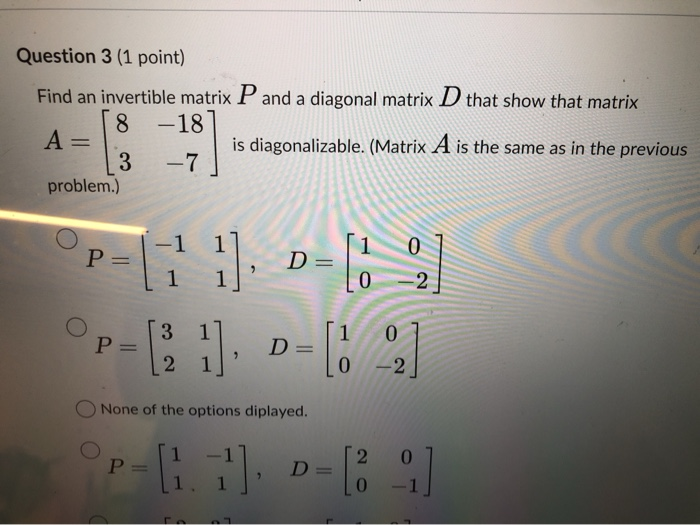Question 3 (1 point) Find an invertible matrix P and a diagonal matrix D that show that matrix 8 -18 A= is diagonalizable. (Matrix A is the same as in the previous 3 - 7 problem.) -1 1 P= 1 1 1]. D=11_, (21]. D= [ ] 1 P= 1 O None of the options diplayed. P-[1.]. D-[ :D

• ### Diagonalise the matrix A that is, find a diagonal matrix D and an invertible matrix P...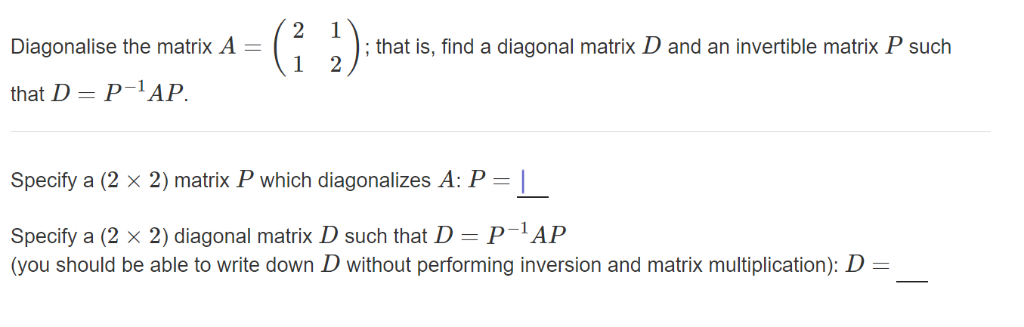Diagonalise the matrix A that is, find a diagonal matrix D and an invertible matrix P such that D = P-IAP Specify a (2 × 2) matrix P which diagonalizes A. P-1 Specify a (2 x 2) diagonal matrix D such that DPAP (you should be able to write down D without performing inversion and matrix multiplication): D-

• ### Let A =[103, 020, 002]. Find an invertible matrix P and a diagonal matrix D such...continues

Let A =[103, 020, 002]. Find an invertible matrix P and a diagonal matrix D such that A =P ? ¹DP.

• ### (1 point) Suppose 11-8 12 -9 A: Find an invertible matrix P and a diagonal matrix...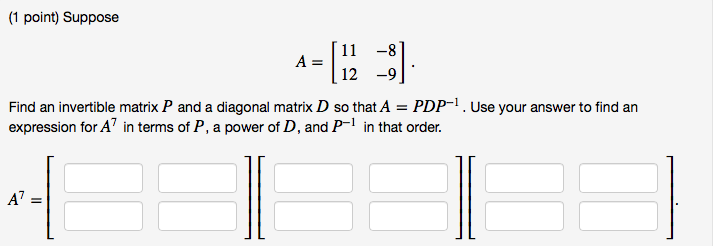(1 point) Suppose 11-8 12 -9 A: Find an invertible matrix P and a diagonal matrix D so that A PDP1.Use your answer to find an expression for A7 in terms of P, a power of D, and P-1 in that order.

• ### (1 point) Suppose A = - (-11, ] Find an invertible matrix P and a diagonal...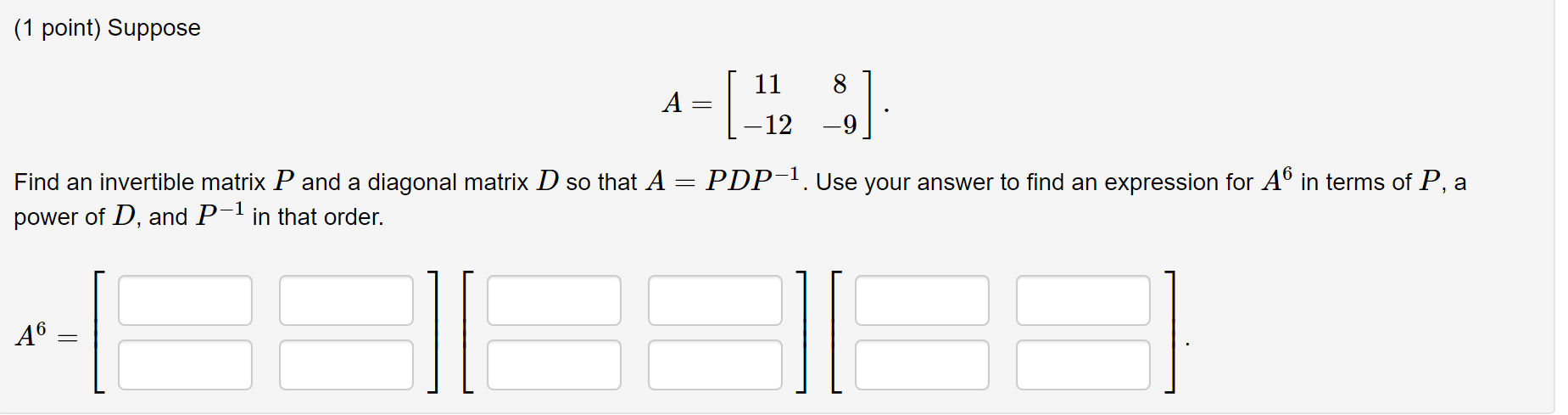(1 point) Suppose A = - (-11, ] Find an invertible matrix P and a diagonal matrix D so that A = PDP-1. Use your answer to find an expression for A6 in terms of P, a power of D, and P-1 in that order. A6 =

Free Homework Help App